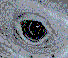Melissa Kaplan's
Herp Care Collection
Last updated January 1, 2014

Health Care: Common Conversion Factors

Terms you are likely to encounter when reading and talking with other herp owners or to your vet.

Melissa Kaplan, 1995

Weight

1 kilogram (kg) = 2.2 pounds (lb) = 1000 g

1 lb = 454 g = .454 kg = 16 oz

kg x 2.2-5 = kg

lb x 0.454 = kg

1 oz = 28.349 g

1 g = 1000 mg

oz = 28.349 = g

g x 0.935 = oz

Liquids

1 oz = 30 milliliters (ml)

1 tbsp = 3 teaspoons (tsp) = 15 ml

1 tsp = 5 ml

2 tbsp = 1 fl oz

4 tbsp = 1/4 c = 2 oz

1 ml = 1 cc

1 cup (c) = 8 fl. oz = 16 tablespoons (tbsp) = 1/2 pt

2 c = 16 fl oz = 1 pt = 1/2 qt

4 qt = 1 gal

1 L = 1000 ml

Length

1 m = 3.281 ft = 39.37 in.

in. x 25.4 = mm

in. x 2.54 = cm

mm x 0.0394 = in.

cm x 0.394 = in.

ft x 0.305 = m

m x 3.281 = ft

m x 1.094 = yd

yd x 0.914 = m

Volume

US fl gal x 0.833 = UK gal

US fl oz x 1.041 = UK fl oz

US fl oz x 29.572 = ml

UK gal x 1.201 = US fl gal

UK fl oz x 0.961 = US fl oz

l (litre) x 1.056 = US fl qt

US fl qt x 0.947 = l (litre)

To calculate gallon capacity of an enclosure:

1. Height x Width x Depth = cubic inches

2. Divide total cubic inches to find gallons:

Total cubic inches divided by 231 c. i. = US gal

Total cubic inches divided by 277.4193 c.i. = UK gal

Temperature

To convert centigrade to Fahrenheit: (C° x 1.8) + 32 = F°

To convert Fahrenheit to centigrade: (F° - 32) x .555 = C°

Notes
Liquid medications are measured in milliliters (ml) or cubic centiliters (cc), with syringes marked accordingly; while measured differently, they equal the same amount of fluid. Markings on sy ringes will vary depending on the total volume of the syringe. Syringes typically used in small animal and reptile care are 1cc, 3cc, 6cc, 12cc, 35cc, and 60cc.

Articles of Related Interest

Web Sites of Related Interest

www.anapsid.org/resources/confac.html

Need to update a veterinary or herp society/rescue listing?

Can't find a vet on my site? Check out these other sites.

 Amphibians Conservation Health Lizards Resources Behavior Crocodilians Herpetology Parent/Teacher Snakes Captivity Education Humor Pet Trade Societies/Rescues Chelonians Food/Feeding Invertebrates Plants Using Internet Clean/Disinfect Green Iguanas & Cyclura Kids Prey Veterinarians Home About Melissa Kaplan CND Lyme Disease Zoonoses Help Support This Site Emergency Preparedness© 1994-2014 Melissa Kaplan or as otherwise noted by other authors of articles on this site